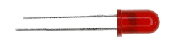﻿ How to Vary the Brightness of an LED# How to Vary the Brightness of an LEDThis is a simple project we're going to do so that we can vary the brightness that an LED gives out. We can make the LED shine very bright or we can make it shine dimly.

To do this, all we do is take a 9-volt battery and connect to a 470Ω resistor which is in series with a 100K potentiometer connected to an LED. We use a protection diode in this circuit so that if the 9-volt battery is inserted the wrong way, the LED can be protected from reverse current. But if you don't have this, it's ok. Just be more careful.

Components Needed

9-Volt Battery
Protection Diode
470Ω resistor (or any resistor within this proximity)
100K Potentiometer
A LED

The schematic of the circuit is shown below:This is now a more real life like representation of the circuit:This is how the circuit works. When the potentiometer is turned all the way to the side where it has a resistance of near 0Ω, the LED shines the brightest because the circuit has the least amount of resistance at this point, so it produces the greatest amount of current, which is fed into the LED. Now as we begin turning the potentiometer to the other side so that its resistance begins to increase, the LED begins getting dimmer and dimmer until it finally goes out completely.

Similarly, we could start with the potentiometer turned the other direction in which it has a resistance of about 100KΩ. At first, the LED gives out no light. However, when we turn the potentiometer so that the resistance decreases, we eventually begin to see the LED light shine dimly. As we keep turning the potentiometer, decreasing its resistance, the LED shines brighter and brighter until it reaches its brightness point when the potentiometer is turned all the way so that its resistance is near 0Ω.

The reason we have the 470Ω resistor in the circuit is so that when the potentiometer is turned so that it outputs almost 0Ω, the LED isn't blown out. If the 470Ω resistor wasn't there, the resistance of the circuit would be extremely low, and so thus a high current would be produced in the current. The current would be too great for the LED to handle and would blow out the LED. The fixed 470Ω resistor is necessary so that the lowest resistance the circuit reaches is at least 470Ω so that too much current isn't produced.

Related Resources